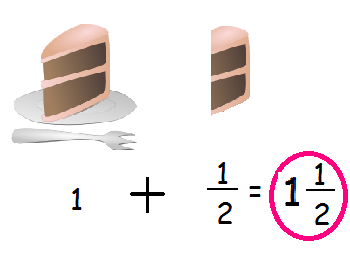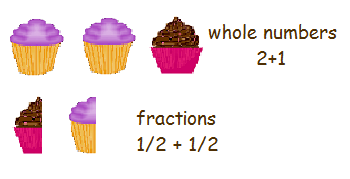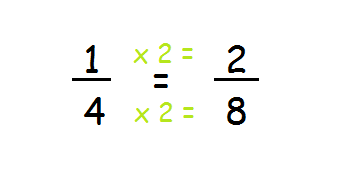Instructor: Emily Hume

Emily is a Reading Specialist and Literacy coach in a public elementary school with a Master's Degree in Elementary Education.

Math is more than just whole numbers; sometimes you will only have a part of a whole, known as a fraction. And sometimes, you can even have a whole and a fraction together. In this lesson, you will learn what compound fractions are and how to add them together.

## What is a Compound Fraction?

It's your birthday! Dad cuts a big piece of cake for you, and one for himself. You gobble yours down and then eat half of your dad's piece! Did you know you have just gobbled down a compound fraction? When you have a number such as 1 1/2, which contains a whole number (such as 1) along with a fraction (1/2), you have a compound fraction, which is frequently called a mixed number.## Adding Compound Fractions with Like Denominators

You take cupcakes to class for your birthday. After your party, you have 1 1/2 chocolate and 2 1/2 vanilla left. So, how many cupcakes do you have left in all? You have to add compound fractions to find out! Here are the steps:

1. Put the whole numbers together and the fractions together, with a plus sign in between (See image: Cupcake Mixed Numbers!)2. Add the whole numbers together: 1 + 2 = 3. Then add the fractions together: 1/2 + 1/2 = 2/2, or 1 whole. (Reminder: When adding fractions, only add the top numbers - the bottom number stays the same!) Add the 3 and the 1 and you have 4 total cupcakes left over!

## Adding Fractions with Unlike Denominators

Because your denominators, or bottom numbers, were the same in your cupcake fractions, adding these fractions was easy! That won't always be the case. Sometimes you will have to find a common denominator, a number that both denominators divide evenly into.

For example, suppose you had 1 1/4 chocolate and 2 1/8 vanilla cupcakes left. The first step is the same: add the whole numbers 1 + 2 = 3. Then you need to find a way to add 1/4 and 1/8. 8 can be divided by 4 and by 8, so it's a good number to use as a common denominator.

To change 1/4, put the 8 on the bottom and divide by 4 (=2). Then take that number and multiply by the top number (2 x 1 = 2). So, 1/4 = 2/8. 2/8 + 1/8 = 3/8. Altogether, you had 3 3/8 total cupcakes leftover.To unlock this lesson you must be a Study.com Member.

### Register to view this lesson

Are you a student or a teacher?

#### See for yourself why 30 million people use Study.com

##### Become a Study.com member and start learning now.
Back
What teachers are saying about Study.com

### Earning College Credit

Did you know… We have over 200 college courses that prepare you to earn credit by exam that is accepted by over 1,500 colleges and universities. You can test out of the first two years of college and save thousands off your degree. Anyone can earn credit-by-exam regardless of age or education level.